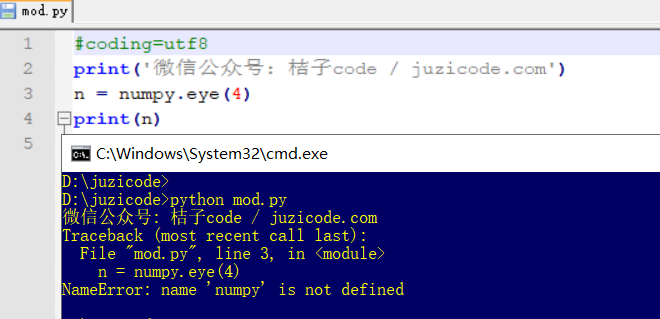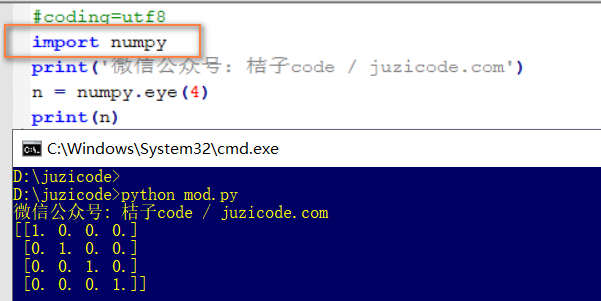# Python error collection: NameError: name ‘numpy’ is not defined

Error message:
Use the numpy.eye() function times NameError: the name ‘numpy’ is not defined

``````D:\juzicode>python mod.py
Traceback (most recent call last):
File "mod.py", line 3, in
n = numpy.eye(4)
NameError: name 'numpy' is not defined``````Possible reasons:
1. Did not import numPY module, but used the functions in numPY module.

Solutions:
1. Import the module NUMpy before calling the function of numpy: